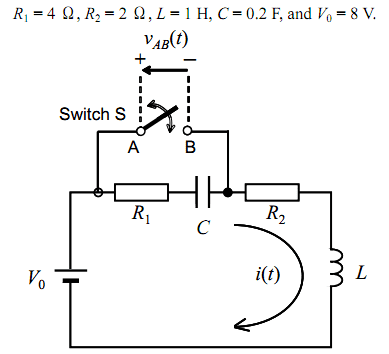# Simple RLC circuit problem

degs2k4

## Homework Statement

I have some difficulties in parts 3 and 4 of the following problem:

(1) A sufficient time has elapsed after closing the switch S, and a steady state is established in the circuit with a constant current I0 flowing. Find the expression for I0.

(2) Then the switch S is opened. At this moment, the time t is defined as t = 0. Find vAB (t = 0+), the voltage appearing between the two switch terminals A and B immediately after opening the switch (t = 0+). vAB (t) is taken positive at terminal A with respect to terminal B.

(3) A sufficient time has elapsed after opening the switch S, and a steady state is established in the circuit. Find vAB (t = ∞), the voltage appearing between the two switch terminals A and B in the steady state.

(4) Find the expressions for i(t) and vAB(t) for t > 0 after opening the switch S at t = 0. Here, i(t) is
the current flowing in the circuit. In this Question (4), use the following circuit constants:## The Attempt at a Solution

(1)　Io = Vo/R
(2)　Vab = Vr1 = Vo(R1 / (R1+R2))
(3)　Vab = Vc = Vo
(4) After KVL, laplace, (using Vo/R as Io of the inductor) I get i(t) as:

i(t) = e^(-t) + 3 e^(-5t)

And to get the voltage between a and b:

Vab(t) = Vr1(t) + Vc(t) = 4 i(t) + 5Int(i(t) dt) = -e^(-t) + 9 e^(-5t)

However, I checked Vab at t=0 and t=infinite, and does not match neither part 2 nor part 3, so something is wrong here...

Thanks

Last edited:

## Answers and Replies

Phrak
Check your work for sign errors. I get, for (3), Vab=V0.

degs2k4
Check your work for sign errors. I get, for (3), Vab=V0.

2 things,

1) How do you get Vab = Vo ? I was wondering between Vo or Vr1...

2) Vab(t) from part 4 still does not match part 3 when t tends to infinity, and can't find any mistake there...

Phrak
Concerning part 3)

For your first question, I'm not sure what your asking, however looking up "battery symbol" on the internet will tell you that the longer line is the positive pole, but you probably knew that.

Vab can't be equal to VR1. Immediately after the switch is opened the current that will flow in the circuit is determined by the capacitor. After a sufficient time, t=infinity the current is steady state. The capacitor blocks all current so the steady state is zero current. No current is flowing in C, therefore no current is flowing in any element, therefore the voltage across all resistors is zero.

e = L di/dt. The current is constantly zero, so zero volts develops across the inductor as well.

degs2k4
Concerning part 3)

For your first question, I'm not sure what your asking, however looking up "battery symbol" on the internet will tell you that the longer line is the positive pole, but you probably knew that.

Vab can't be equal to VR1. Immediately after the switch is opened the current that will flow in the circuit is determined by the capacitor. After a sufficient time, t=infinity the current is steady state. The capacitor blocks all current so the steady state is zero current. No current is flowing in C, therefore no current is flowing in any element, therefore the voltage across all resistors is zero.

e = L di/dt. The current is constantly zero, so zero volts develops across the inductor as well.

Regarding part 3, what I want to ask is :
Since there is a resistor connected in series before the capacitor, there is a voltage drop Vr1, so we can say that the applied voltage to the capacitor is Vr1.
A capacitor charges until reaching the applied voltage, so I was wondering whether the final voltage of the capacitor in steady state was either Vr1 or Vo...

I am sorry for not being clear...

Phrak
In part 3, a current has been established. Then we talk about what is going on an infinite amount of time after erasing the switch from the schematic.

The L and C are not going to resonate forever because you have resistors dissipating energy. This means the current goes to zero. With zero current, VR1 must be zero (V=IR). You should convince yourself that all the voltage of the source appears across the capacitor.

Does this help?

degs2k4
In part 3, a current has been established. Then we talk about what is going on an infinite amount of time after erasing the switch from the schematic.

The L and C are not going to resonate forever because you have resistors dissipating energy. This means the current goes to zero. With zero current, VR1 must be zero (V=IR). You should convince yourself that all the voltage of the source appears across the capacitor.

Does this help?

I see! Clear now, thanks!

Still stuck on part (4), couldn't find any errors but I am going to check it again...

Thanks Phrak

Phrak
Good deal. I don't know laplace anyway, and I'd have to do it the hard way.

Homework Helper
Because of the integration, you need to add a constant to Q(t).

ehild

degs2k4
Because of the integration, you need to add a constant to Q(t).

ehild

And such constant (I guess you speak about the initial charge of the capacitor) is zero right ?

Homework Helper
Yes.

ehild

degs2k4
Yes.

ehild

That is why I didn't add such constant, since it is zero, 0/s will be zero... so I think that is not the mistake...

Homework Helper
Write the time dependence of Q. If it contains only exponential terms it is not zero at t=0.

ehild